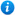# Calculating Angles on a Straight Line - Year 5

Calculating Angles on a Straight Line - Year 5

Year 5 - Geometry - properties of shapes

Pupils should be taught to:

• Identify angles at a point on a straight line
In this geometry teaching resource, pupils will learn how many degrees are in a straight angle and how to work out missing angles using their existing knowledge of angle facts. This lesson covers the year 5 curriculum objective listed above and supports the White Rose small steps guidance for year 5 - Summer - Block 2 - Geometry - properties of shapes. Content includes:
• Angles on a straight line recap
• How to calculate missing angles on a straight line explanation
• Calculating missing angles on a straight line class activity
• 3 differentiated worksheets with answers

'Calculating Angles on a Straight Line - Year 5' is completely editable giving teachers the freedom to adapt the resource to suit their individual teaching needs.

Click on the images from the PowerPoint presentation to view the resource in more detail.

Our Price : £2.50 / 3 Credits